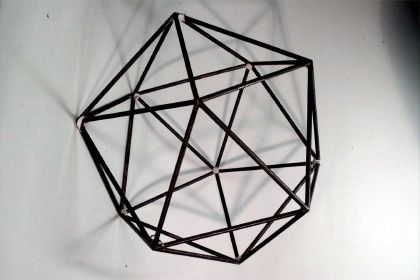Göttingen Collection of Mathematical Models and Instruments

# Icosahedron

### Model 703Category: B II 28

### Description

Icosahedron (brass pipe)

The icosahedron has 20 equilateral triangles as faces. It has twelve vertices and 30 edges. At each vertex five triangles are meeting.

The icosahedron is one of the five Platonic solids. A Platonic solid is a regular, convex polyhedron. The faces of a Platonic solid are congruent, regular polygons. At each vertex the same number of faces are meeting, see also model 411.

The five Platonic solids are Tetrahedron, Hexahedron (Cube), Octahedron, Dodecahedron and Icosahedron.

Showcase of this model is Case number 21

### References

Separataband M4 im Mathematischen Institut p. 231.

Separataband M5 im Mathematischen Institut p. 41, 45.

Separataband M5 im Mathematischen Institut p. 4.